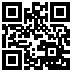## 20100712

### Education research: introductory astronomy laboratory student promptness (Cuesta College, Fall Semester 2009-Spring Semester 2010)Astronomy 210L, Fall Semester 2009-Spring Semester 2010
Cuesta College, San Luis Obispo, CA

Students are assigned to read online articles on current astronomy events, and take a short current events quiz during the first 10 minutes of lab. (This motivates students to show up promptly to lab, as the time cut-off for the quiz is strictly enforced!)

The following summarizes the statistical analysis involved in counting the students that come later than 10 minutes into lab (and thus miss taking the current events quiz).

Student attendance status was determined for each lab using the following IF() function:
=IF(COUNTA(Labscore)>0,IF(COUNTA(Quizscore)>0,"here","late"),"absent")
• So students with no lab scores entered are marked "absent."
• Of students with a recorded lab score, those with no current events quiz score recorded are marked "late."
• Of students with a recorded lab score, those with a recorded current events quiz score recorded are marked "here."
The totals of "absent," "late," and "here" counts are divided by the product of the total number of labs (13) times the total number of students:
Absent rate = ("absent" tag count)/(number of labs)*(number of students),
and similarly to calculate the late and full attendance rates.

For the past two semester, the average current events quiz scores were recorded, along with student attendance rates:
`Cuesta CollegeAstronomy 210L Fall Semester 2010 sections 70178, 70186, 70200(N = 66)Current events quiz average score: 76.6% +/- 20.3%Absent rate:                       10.7%Late (missed current events quiz):  1.9%Full attendance rate:              87.4%Astronomy 210L Spring Semester 2010 sections 30678, 30679, 30680, 30682(N = 86)Current events quiz average score: 77.3% +/- 24.1%Absent rate:                       12.0%Late (missed current events quiz):  2.9%Full attendance rate:              85.1%`
The average current events quiz scores, along with attendance rates are comparable between these two semesters.

Notably, the number of students who come to lab more than 10 minutes late (and thus miss taking the current events quiz) ranged from an average of one late student per week (66*0.019 = 1.254 in Fall 2010) to 2.5 late students per week (86*0.029 = 2.494 in Spring 2010), or an overall rate of 2.0 late students per week (weighted average of (66*1.254 + 86*2.494)/(66 + 86)).

There were no statistics on on-time versus late students compiled in this course before current events quizzes were first implemented (in Fall 2006), so there is no directly comparable control (no entry quiz) population to compare with in order to determine the effect of current events quizzes on student promptness. This coming semester (Fall 2010) informal observations of tardiness in introductory college physics laboratory and general survey geology laboratory will be made in the same institution (Cuesta College, San Luis Obispo, CA).